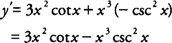## Trigonometric Function Differentiation

The six trigonometric functions also have differentiation formulas that can be used in application problems of the derivative. The rules are summarized as follows:

1. If f( x) = sin x, then f′( x) = cos x

2. If f( x) = cos x, then f′( x) = −sin x

3. If f( x) = tan x, then f′( x) = sec 2 x

4. If f( x) = cot x, then f′( x) = −csc 2 x.

5. If f( x) = sec x, then f′( x) = sec x tan x

6. If f( x) = csc x, then f′( x) = −csc x cot x

Note that rules (3) to (6) can be proven using the quotient rule along with the given function expressed in terms of the sine and cosine functions, as illustrated in the following example.

Example 1: Use the definition of the tangent function and the quotient rule to prove if f( x) = tan x, than f′( x) = sec 2 x.Example 2: Find y′ if y = x 3 cot x.Example 3: Findif f( x) = 5 sin x + cos xExample 4: Find the slope of the tangent line to the curve y = sin x at the point (π/2,1)

Because the slope of the tangent line to a curve is the derivative, you find that y′ = cos x; hence, at (π/2,1), y′ = cos π/2 = 0, and the tangent line has a slope 0 at the point (π/2,1). Note that the geometric interpretation of this result is that the tangent line is horizontal at this point on the graph of y = sin x.